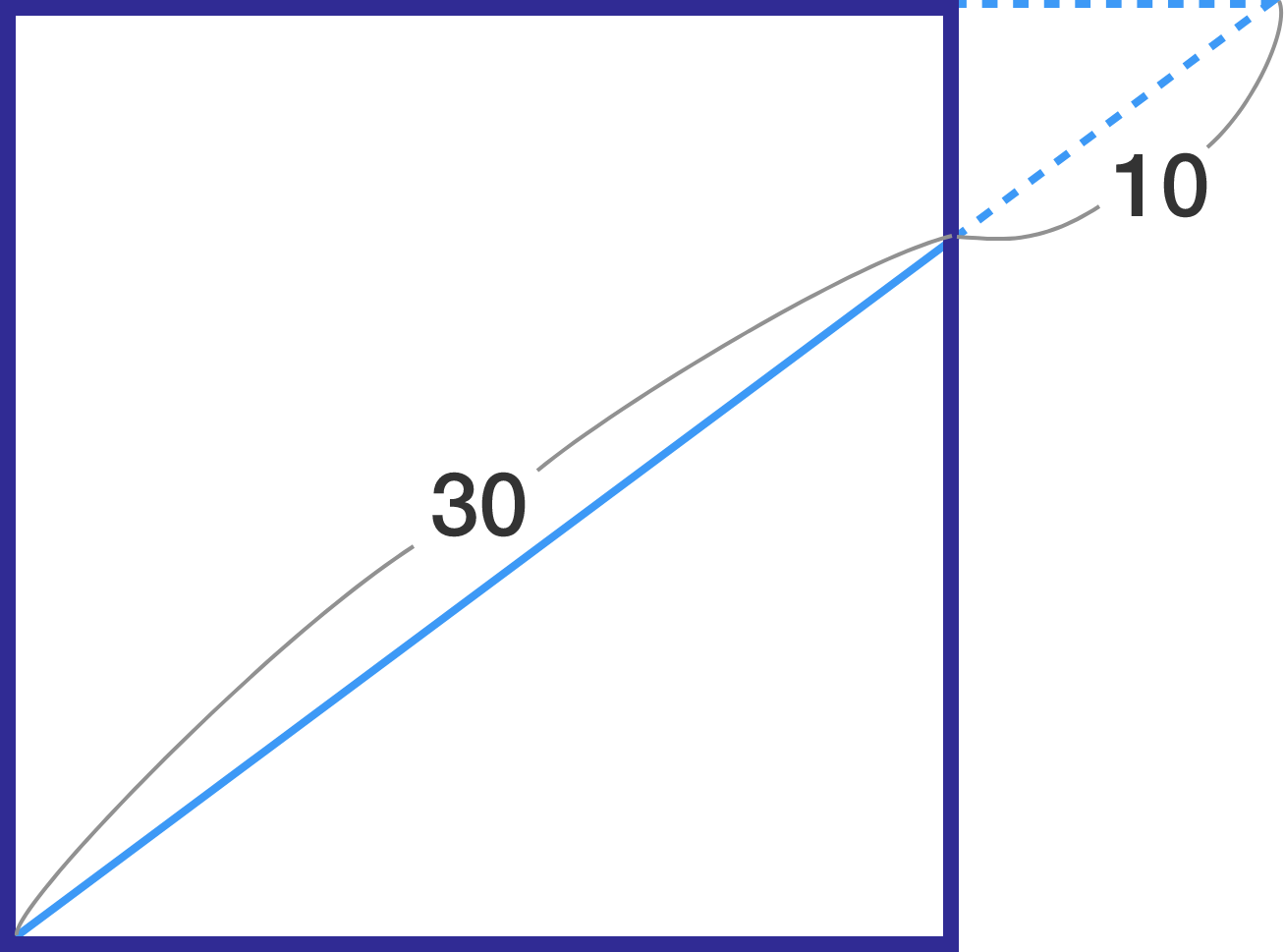# Similar triangles

Geometry Level 3

In the diagram below, the top side of a square is extended to the right, the end of which is then connected to the lower left vertex of the square. This line segment is divided into two parts: the part with length 30 inside the square and the part with length 10 outside.

What is the side length of the square?×